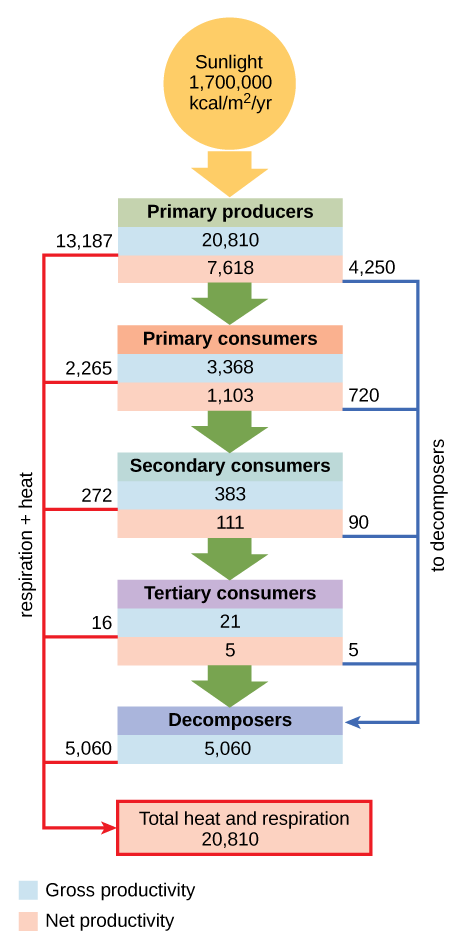# 46.1D: Modeling Ecosystem Dynamics

•• Boundless
• Boundless
$$\newcommand{\vecs}{\overset { \rightharpoonup} {\mathbf{#1}} }$$ $$\newcommand{\vecd}{\overset{-\!-\!\rightharpoonup}{\vphantom{a}\smash {#1}}}$$$$\newcommand{\id}{\mathrm{id}}$$ $$\newcommand{\Span}{\mathrm{span}}$$ $$\newcommand{\kernel}{\mathrm{null}\,}$$ $$\newcommand{\range}{\mathrm{range}\,}$$ $$\newcommand{\RealPart}{\mathrm{Re}}$$ $$\newcommand{\ImaginaryPart}{\mathrm{Im}}$$ $$\newcommand{\Argument}{\mathrm{Arg}}$$ $$\newcommand{\norm}{\| #1 \|}$$ $$\newcommand{\inner}{\langle #1, #2 \rangle}$$ $$\newcommand{\Span}{\mathrm{span}}$$ $$\newcommand{\id}{\mathrm{id}}$$ $$\newcommand{\Span}{\mathrm{span}}$$ $$\newcommand{\kernel}{\mathrm{null}\,}$$ $$\newcommand{\range}{\mathrm{range}\,}$$ $$\newcommand{\RealPart}{\mathrm{Re}}$$ $$\newcommand{\ImaginaryPart}{\mathrm{Im}}$$ $$\newcommand{\Argument}{\mathrm{Arg}}$$ $$\newcommand{\norm}{\| #1 \|}$$ $$\newcommand{\inner}{\langle #1, #2 \rangle}$$ $$\newcommand{\Span}{\mathrm{span}}$$

##### Learning Objectives
• Compare and contrast conceptual, analytical, and simulation models of ecosystem dynamics

## Conceptual models

Conceptual models are useful for describing ecosystem structure and dynamics and for demonstrating the relationships between different organisms in a community and their environment. Conceptual models are usually depicted graphically as flow charts. The organisms and their resources are grouped into specific compartments with arrows showing the relationship and transfer of energy or nutrients between them. These diagrams are sometimes called compartment models.Figure $$\PageIndex{1}$$: Conceptual model of energy: This conceptual model shows the flow of energy through a spring ecosystem in Silver Springs, Florida. Notice that the energy decreases with each increase in trophic level. Conceptual models are useful for describing ecosystem structure, but are limited by their poor prediction of ecosystem changes.

To model the cycling of mineral nutrients, organic and inorganic nutrients are subdivided into those that are bioavailable (ready to be incorporated into biological macromolecules) and those that are not. For example, in a terrestrial ecosystem near a deposit of coal, carbon will be available to the plants of this ecosystem as carbon dioxide gas in a short-term period, not from the carbon-rich coal itself. However, over a longer period, microorganisms capable of digesting coal will incorporate its carbon or release it as natural gas (methane, CH4), changing this unavailable organic source into an available one.

Human combustion of fossil fuels accelerates this conversion by releasing large amounts of carbon dioxide into the atmosphere, which may be a large contributor to the rise of the atmospheric carbon dioxide levels in the industrial age. The carbon dioxide released from burning fossil fuels is produced faster than photosynthetic organisms can use it, while the number of photosynthetic trees have decreased because of worldwide deforestation. Most scientists agree that high atmospheric carbon dioxide is a major cause of global climate change.

## Analytical and simulation models

Conceptual models are limited; they poorly predict the consequences of changes in ecosystem species and/or environment. Ecosystems are dynamic entities that are subject to a variety of abiotic and biotic disturbances. In these cases, scientists often use analytical or simulation models. These models predict how ecosystems recover from disturbances, returning to a state of equilibrium. As most ecosystems are subject to periodic disturbances and are often in a state of change, they are usually either moving toward or away from multiple equilibrium states. Since human impact can greatly and rapidly alter the species content and habitat of an ecosystem, it is crucial for scientists to develop models that predict how ecosystems respond to these changes.

## Analytical models

Analytical models generally work best when dealing with relatively-simple, linear systems; specifically, those that can be accurately described by a set of mathematical equations whose behavior is well known. They are mathematically complex models that are good at predicting components of ecosystems such as food chains. However, their accuracy is limited by their simplification of complex ecosystems.

## Simulation models

Like analytical models, simulation models use complex algorithms to predict ecosystem dynamics. However, sophisticated computer programs have enabled simulation models to predict responses in complex ecosystems. Simulation models use numerical techniques to solve problems for which analytic solutions are impractical or impossible. These kinds of models tend to be more widely used. They are generally considered more ecologically realistic, while analytic models are valued for their mathematical elegance and explanatory power. These simulations are considered to be the most accurate and predictive of ecosystem dynamics.

## Key Points

• Conceptual models are often flow charts that demonstrate the relationships between different organisms in a community and their environment, including the transfer of energy and nutrients.
• Analytical models use mathematical equations to predict and describe simple, linear components of ecosystems, such as food chains.
• Simulation models use computer algorithms to predict ecosystem dynamics; they are considered the most ecologically-realistic and accurate.

## Key Terms

• conceptual model: a model that is represented by conceptual representations of the relationships between different organisms in a community and their environment
• analytical model: a model that works best when dealing with relatively simple (often linear) systems, specifically those that can be accurately described by a set of mathematical equations whose behavior is well known
• simulation model: a model that utilizes mathematical algorithms to predict complex responses in ecosystem dynamics